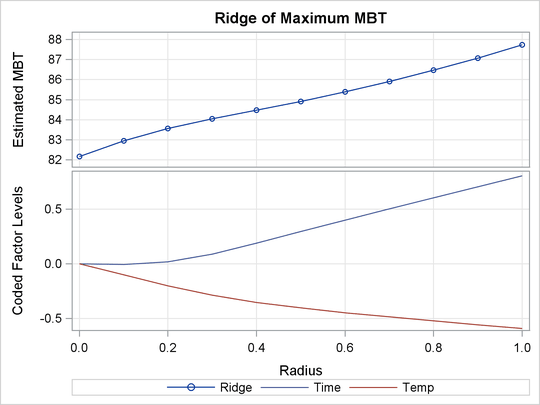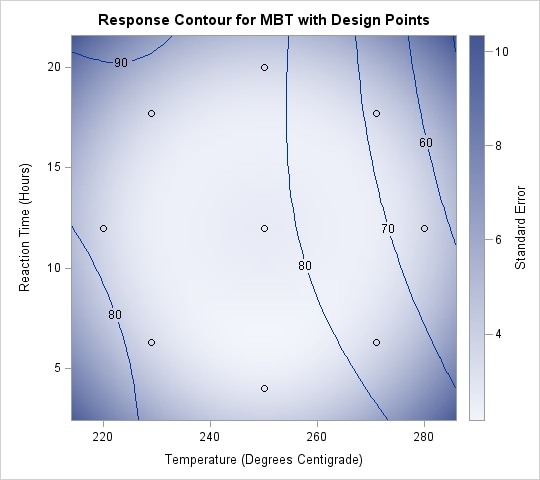Example 81.1 A Saddle Surface Response Using Ridge Analysis

Myers (1976) analyzes an experiment reported by Frankel (1961) aimed at maximizing the yield of mercaptobenzothiazole (MBT) by varying processing time and temperature. Myers (1976) uses a two-factor model in which the estimated surface does not have a unique optimum. A ridge analysis is used to determine the region in which the optimum lies. The objective is to find the settings of time and temperature in the processing of a chemical that maximize the yield. The following statements produce Output 81.1.1 through Output 81.1.6:

```data d;
input Time Temp MBT;
label Time = "Reaction Time (Hours)"
MBT  = "Percent Yield Mercaptobenzothiazole";
datalines;
4.0   250   83.8
20.0   250   81.7
12.0   250   82.4
12.0   250   82.9
12.0   220   84.7
12.0   280   57.9
12.0   250   81.2
6.3   229   81.3
6.3   271   83.1
17.7   229   85.3
17.7   271   72.7
4.0   250   82.0
;
```
```ods graphics on;
proc rsreg data=d plots=(ridge surface);
model MBT=Time Temp / lackfit;
ridge max;
run;
ods graphics off;
```

Output 81.1.1 displays the coding coefficients for the transformation of the independent variables to lie between –1 and 1 and some simple statistics for the response variable.

Output 81.1.1: Coding and Response Variable Information

The RSREG Procedure

Coding Coefficients for the Independent
Variables
Factor Subtracted off Divided by
Time 12.000000 8.000000
Temp 250.000000 30.000000

Response Surface for Variable MBT: Percent
Yield Mercaptobenzothiazole
Response Mean 79.916667
Root MSE 4.615964
R-Square 0.8003
Coefficient of Variation 5.7760

Output 81.1.2 shows that the lack of fit for the model is highly significant. Since the quadratic model does not fit the data very well, firm statements about the underlying process should not be based only on the current analysis. Note from the analysis of variance for the model that the test for the time factor is not significant. If further experimentation is undertaken, it might be best to fix `Time` at a moderate to high value and to concentrate on the effect of temperature. In the actual experiment discussed here, extra runs were made that confirmed the results of the following analysis.

Output 81.1.2: Analyses of Variance

Regression DF Type I Sum of Squares R-Square F Value Pr > F
Linear 2 313.585803 0.4899 7.36 0.0243
Quadratic 2 146.768144 0.2293 3.44 0.1009
Crossproduct 1 51.840000 0.0810 2.43 0.1698
Total Model 5 512.193947 0.8003 4.81 0.0410

Residual DF Sum of Squares Mean Square F Value Pr > F
Lack of Fit 3 124.696053 41.565351 39.63 0.0065
Pure Error 3 3.146667 1.048889
Total Error 6 127.842720 21.307120

Parameter DF Estimate Standard Error t Value Pr > |t| Parameter Estimate
from Coded Data
Intercept 1 -545.867976 277.145373 -1.97 0.0964 82.173110
Time 1 6.872863 5.004928 1.37 0.2188 -1.014287
Temp 1 4.989743 2.165839 2.30 0.0608 -8.676768
Time*Time 1 0.021631 0.056784 0.38 0.7164 1.384394
Temp*Time 1 -0.030075 0.019281 -1.56 0.1698 -7.218045
Temp*Temp 1 -0.009836 0.004304 -2.29 0.0623 -8.852519

Factor DF Sum of Squares Mean Square F Value Pr > F Label
Time 3 61.290957 20.430319 0.96 0.4704 Reaction Time (Hours)
Temp 3 461.250925 153.750308 7.22 0.0205 Temperature (Degrees Centigrade)

The canonical analysis (Output 81.1.3) indicates that the predicted response surface is shaped like a saddle. The eigenvalue of 2.5 shows that the valley orientation of the saddle is less curved than the hill orientation, with an eigenvalue of –9.99. The coefficients of the associated eigenvectors show that the valley is more aligned with `Time` and the hill with `Temp`. Because the canonical analysis resulted in a saddle point, the estimated surface does not have a unique optimum.

Output 81.1.3: Canonical Analysis

Factor Critical Value Label
Coded Uncoded
Time -0.441758 8.465935 Reaction Time (Hours)
Temp -0.309976 240.700718 Temperature (Degrees Centigrade)

Eigenvalues Eigenvectors
Time Temp
2.528816 0.953223 -0.302267
-9.996940 0.302267 0.953223

However, the ridge analysis in Output 81.1.4 and the ridge plot in Output 81.1.5 indicate that maximum yields result from relatively high reaction times and low temperatures. A contour plot of the predicted response surface, shown in Output 81.1.6, confirms this conclusion.

Output 81.1.4: Ridge Analysis

Estimated Ridge of Maximum Response for Variable MBT: Percent Yield Mercaptobenzothiazole
Coded Radius Estimated Response Standard Error Uncoded Factor Values
Time Temp
0.0 82.173110 2.665023 12.000000 250.000000
0.1 82.952909 2.648671 11.964493 247.002956
0.2 83.558260 2.602270 12.142790 244.023941
0.3 84.037098 2.533296 12.704153 241.396084
0.4 84.470454 2.457836 13.517555 239.435227
0.5 84.914099 2.404616 14.370977 237.919138
0.6 85.390012 2.410981 15.212247 236.624811
0.7 85.906767 2.516619 16.037822 235.449230
0.8 86.468277 2.752355 16.850813 234.344204
0.9 87.076587 3.130961 17.654321 233.284652
1.0 87.732874 3.648568 18.450682 232.256238

Output 81.1.5: Ridge Plot of Predicted Response SurfaceOutput 81.1.6: Contour Plot of Predicted Response Surface